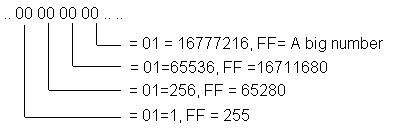>>>> 3
In order to change a save game file, you need to know how the numbers are stored in the files.

Hexadecimal, which is abbreviated as hex when used in computer terms, is a different counting base.
Usually us humans use base -10. This is that from 1 to 10 there is 10 numbers eg.
1 2 3 4 5 6 7 8 9 10

But hexadecimal is base - 16. To count to 10 you go through 16 numbers eg.
1 2 3 4 5 6 7 8 9 a b c d e f 10

As computers use Binary, which is base - 2, eg.
1 10
this is very messy to look at, it would be converted to hexadecimal to make it easier to program in machine code.

DOS only uses one byte per character, one byte equalling 8 binary spaces or 0000 0000. So the highest number you can show in binary is 1111 1111 which equals FF in hexadecimal.
This is only effective up to the number 255 which is what the above binary and hexadecimal numbers equal.

Games store numbers in hexadecimal format instead of character format the idea being that a number like 255 stored as hexadecimal uses less disk space than as characters.
For example, 255 stored as hexadecimal would be:
FF
which equals a single character

But if you stored 255 as characters it would take up 3 bytes:
.. 32 35 35 .. .. 2 5 5
(hex characters)

Note: If you are already computer competent, then you may wonder why I am referring to FF as meaning 255, while when RAM is concerned 256 is used instead; this is because mathematically FF does equal 255, but in computer terms, if you include 00 as equalling one unit, then FF would make a total of 256 separate units.

You can't have more than double digits with a hex number, so what if you need a number higher than 255? (or FF in hex)
Well there is a tricky formula used to store higher numbers in hex, here is a rough diagram
showing it: